# DeepFM详细流程解析及建模演示

## · 流程解析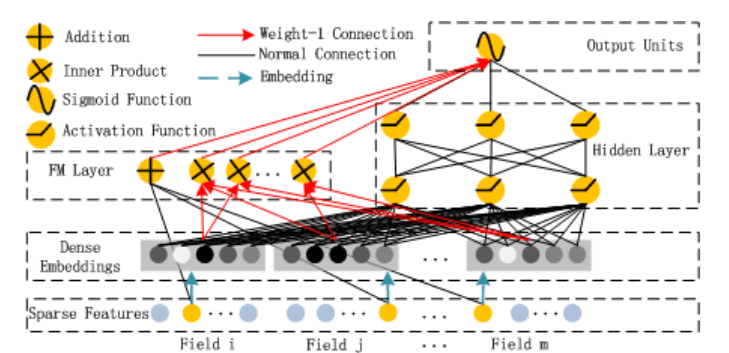### 1、Sparse Feature框框

Sparse Feature是指离散型变量。比如现在我有数据：xx公司每个员工的姓名、年龄、岗位、收入的表格，那么年龄和岗位就属于离散型变量，而收入则称为连续型变量。这从字面意思也能够理解。

### 2、Dense Embedding框框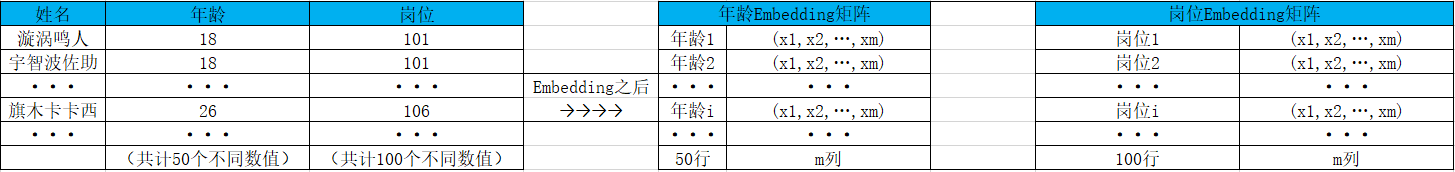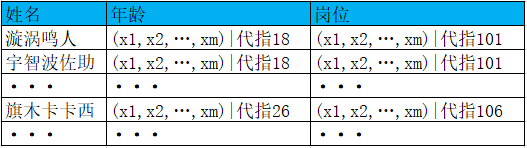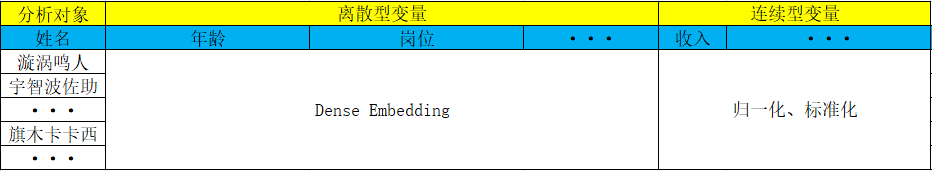(x1,x2,...,xm,x1,x2,...,xm,...,···,x1,x2,...,xn)

x1,x2,...,xm 表示他的年龄的Embedding向量，x1,x2,...,xm 表示他的岗位的Embedding向量，... 表示他的其他属性的Embedding向量，x1,x2,...,xn 表示他的收入等其他连续型特征的归一化或标准化后的值。

### 3、FM Layer框框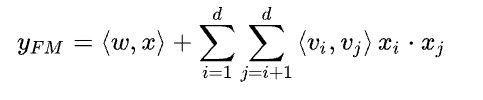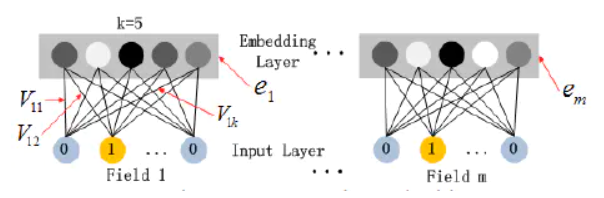### 5、Outputs Units框框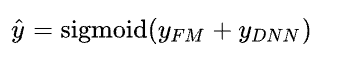## · 建模演示

### 1、DeepFM作分类

``````import pandas as pd
from sklearn.metrics import log_loss, roc_auc_score    # 模型评估指标：对数损失函数、ROC_AUC
from sklearn.model_selection import train_test_split   # 模型训练集和测试集划分
from sklearn.preprocessing import LabelEncoder, MinMaxScaler  # 数据预处理：标签编码、最大最小标准化

from deepctr.models import *   # CTR全部模型（也可只导入DeepFM）
from deepctr.feature_column import SparseFeat, DenseFeat, get_feature_names  # 特征处理
``````
``````data = pd.read_csv('./criteo_sample.txt')

sparse_features = ['C' + str(i) for i in range(1, 27)]
dense_features = ['I' + str(i) for i in range(1, 14)]

# 缺失值填充
data[sparse_features] = data[sparse_features].fillna('-1', )
data[dense_features] = data[dense_features].fillna(0, )

# 拟合目标为数据的标签列
target = ['label']
data
``````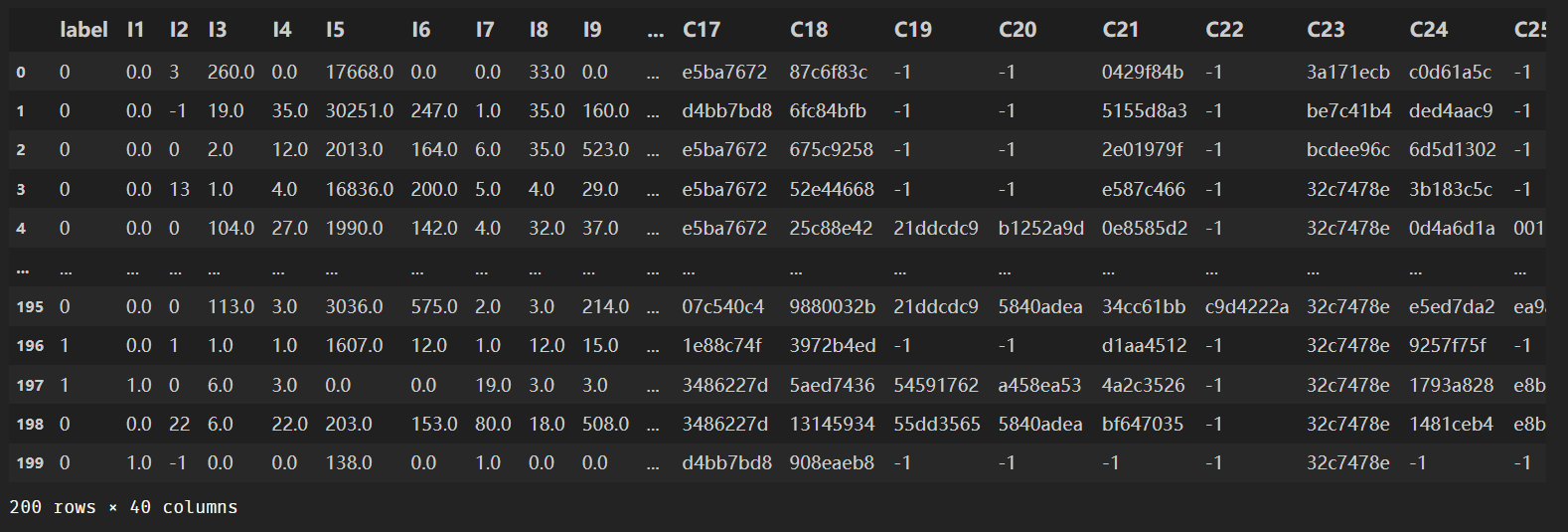``````# 1.Label Encoding for sparse features,and do simple Transformation for dense features
for feat in sparse_features:
# 对离散特征先作了一个标签编码（自然数编码）
lbe = LabelEncoder()
data[feat] = lbe.fit_transform(data[feat])
# 对连续特征作最大最小标准化
mms = MinMaxScaler(feature_range=(0, 1))
data[dense_features] = mms.fit_transform(data[dense_features])
data
``````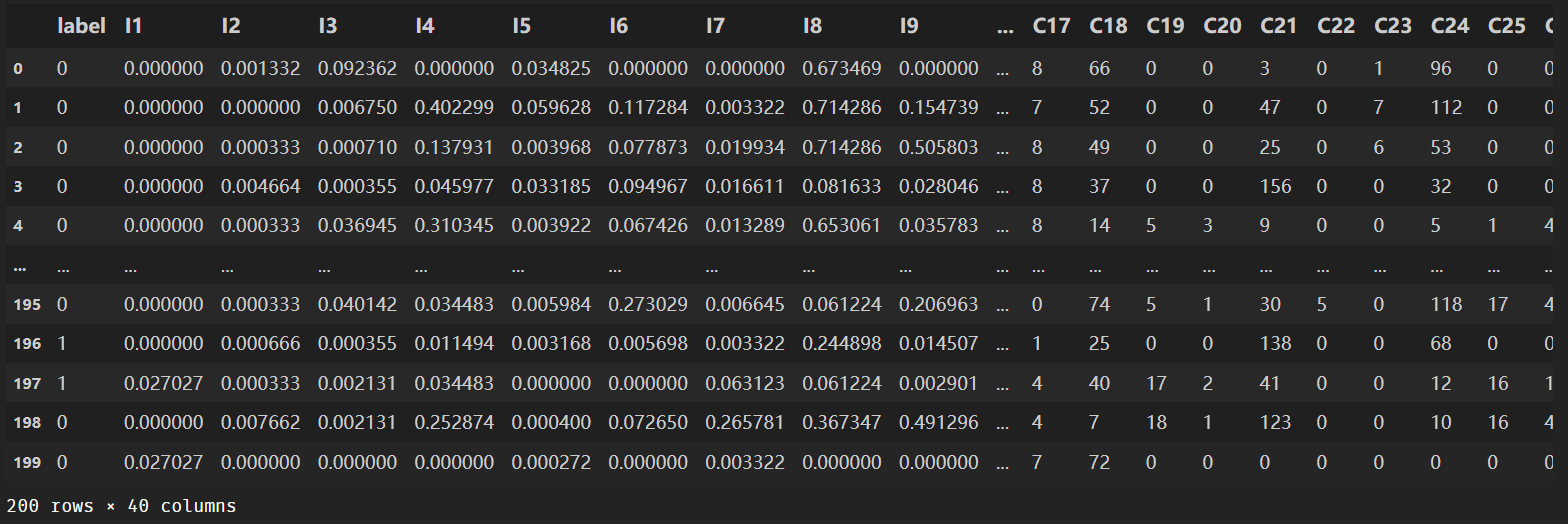``````# 2.count #unique features for each sparse field,and record dense feature field name

fixlen_feature_columns = [SparseFeat(feat, vocabulary_size=data[feat].max() + 1, embedding_dim=4)
for i, feat in enumerate(sparse_features)] + [DenseFeat(feat, 1, )
for feat in dense_features]
# vocabulary_size：embedding向量矩阵的行数；embedding_dim：embedding向量的维数
dnn_feature_columns = fixlen_feature_columns
linear_feature_columns = fixlen_feature_columns

feature_names = get_feature_names(linear_feature_columns + dnn_feature_columns)
``````

``````# 3.generate input data for model

train, test = train_test_split(data, test_size=0.2, random_state=2020)
train_model_input = {name: train[name] for name in feature_names}
test_model_input = {name: test[name] for name in feature_names}
``````
``````# 4.Define Model,train,predict and evaluate
metrics=['binary_crossentropy'], )
history = model.fit(train_model_input, train[target].values,
batch_size=256, epochs=10, verbose=2, validation_split=0.2, )
# batch_size：批梯度下降的每次样本数；epochs：迭代次数；validation_split：验证集比例；verbose：输出训练日志
pred_ans = model.predict(test_model_input, batch_size=256)
print("test LogLoss", round(log_loss(test[target].values, pred_ans), 4))
print("test AUC", round(roc_auc_score(test[target].values, pred_ans), 4))
``````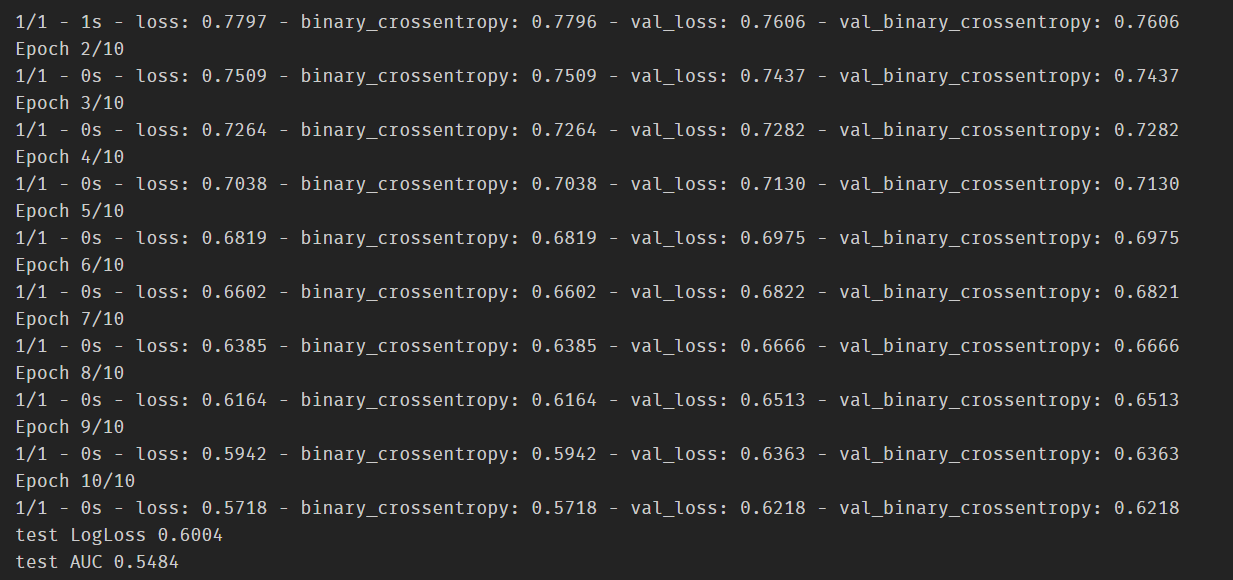``````pd.DataFrame(pred_ans)
``````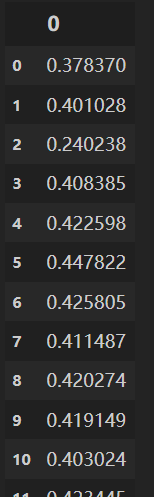pred_ans表示的是预测该样本为正类的概率值，其顺序与数据输入顺序一致。

### 2、DeepFM作回归

``````import pandas as pd
from sklearn.metrics import mean_squared_error
from sklearn.model_selection import train_test_split
from sklearn.preprocessing import LabelEncoder

from deepctr.models import DeepFM
from deepctr.feature_column import SparseFeat,get_feature_names
``````
``````data = pd.read_csv("./movielens_sample.txt")
data
``````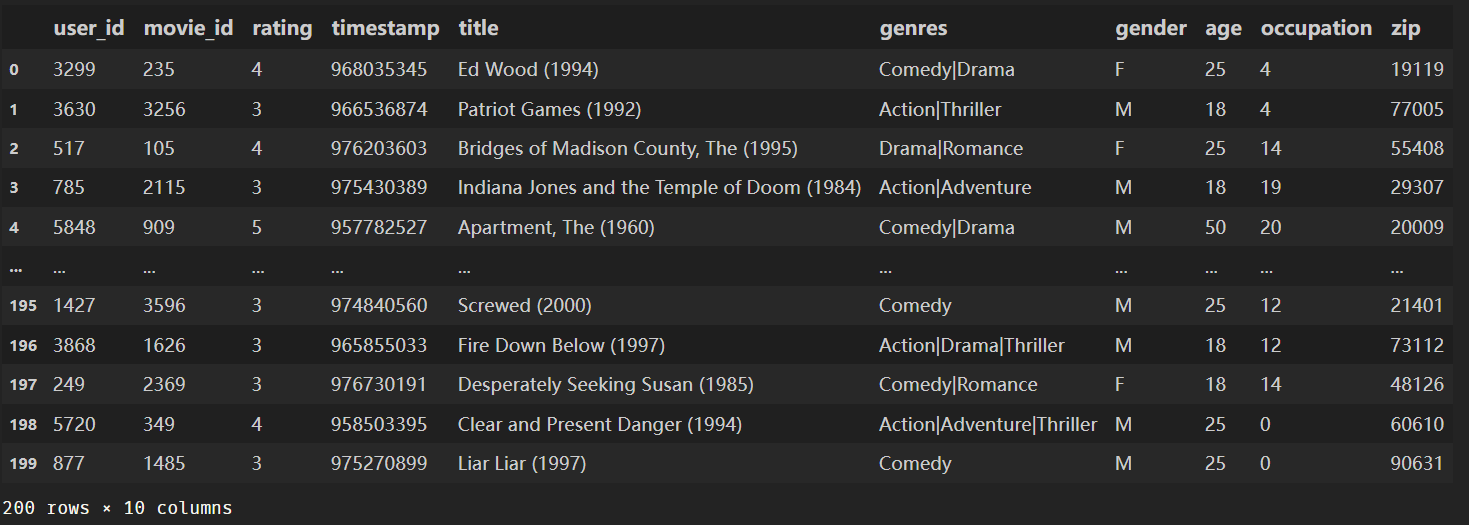``````sparse_features = ["movie_id", "user_id", "gender", "age", "occupation", "zip"]
target = ['rating']
``````
``````# 1.Label Encoding for sparse features,and do simple Transformation for dense features
for feat in sparse_features:
lbe = LabelEncoder()
data[feat] = lbe.fit_transform(data[feat])
``````
``````# 2.count #unique features for each sparse field
fixlen_feature_columns = [SparseFeat(feat, data[feat].max() + 1,embedding_dim=4)
for feat in sparse_features]
linear_feature_columns = fixlen_feature_columns
dnn_feature_columns = fixlen_feature_columns
feature_names = get_feature_names(linear_feature_columns + dnn_feature_columns)
data
````````````# 3.generate input data for model
train, test = train_test_split(data, test_size=0.2, random_state=2020)
train_model_input = {name:train[name].values for name in feature_names}
test_model_input = {name:test[name].values for name in feature_names}
``````
``````# 4.Define Model,train,predict and evaluate

history = model.fit(train_model_input, train[target].values,
batch_size=256, epochs=10, verbose=2, validation_split=0.2, )
pred_ans = model.predict(test_model_input, batch_size=256)
print("test MSE", round(mean_squared_error(test[target].values, pred_ans), 4))
``````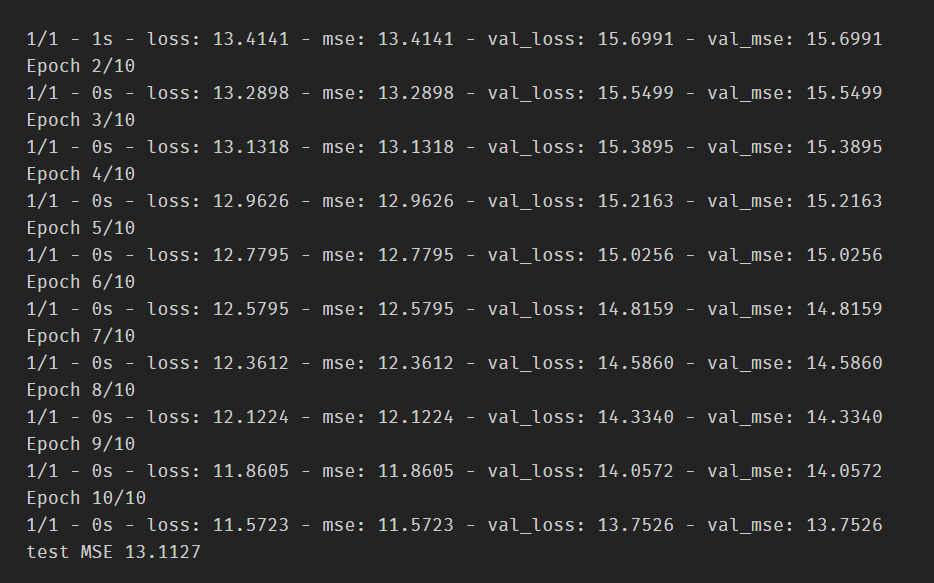``````pd.DataFrame(pred_ans).head()
``````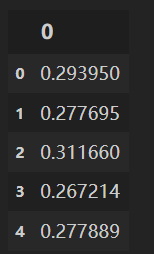pred_ans表示的是预测该样本拟合的目标函数值，其顺序与数据输入顺序一致。

### 3、User和Item的Embedding向量提取

``````feature_columns = [SparseFeat('user_id',120,),SparseFeat('movie_id',60,)]

def get_embedding_weights(dnn_feature_columns,model):
embedding_dict = {}
for fc in dnn_feature_columns:
if hasattr(fc,'embedding_name'):
if fc.embedding_name is not None:
name = fc.embedding_name
else:
name = fc.name
embedding_dict[name] = model.get_layer("sparse_emb_"+name).get_weights()
return embedding_dict

embedding_dict = get_embedding_weights(feature_columns,model)

user_id_emb = embedding_dict['user_id']
movie_id_emb = embedding_dict['movie_id']
``````
``````pd.DataFrame(user_id_emb)
``````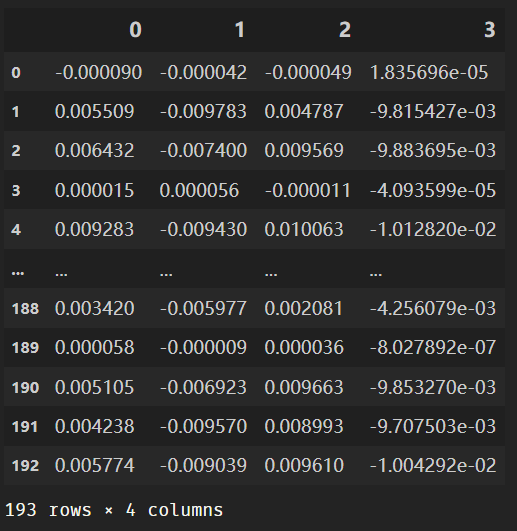``````pd.DataFrame(movie_id_emb)
``````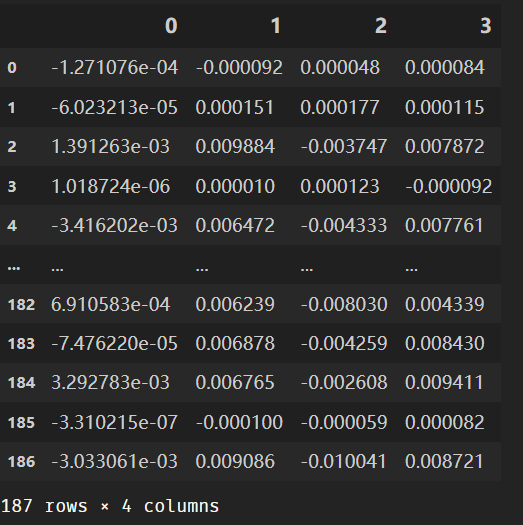## · DeepFM的优点

DeepFM的FM和Deep部分共享相同的输入，可以提高训练效率，不需要额外的特征工程，用FM建模低阶的特征组合，用DNN建模高阶的特征组合，因此可以同时从原始特征中学习到高阶和低阶的特征交互。

DeepFM也是被广泛应用在点击率预测中的深度学习模型，主要关注如何学习用户行为(user behavior)的组合特征(feature interactions)，从而最大化推荐系统CTR。

Original: https://blog.csdn.net/Jeremiah_/article/details/120740877
Author: Eureka丶
Title: DeepFM详细流程解析及建模演示

# 1 问题分析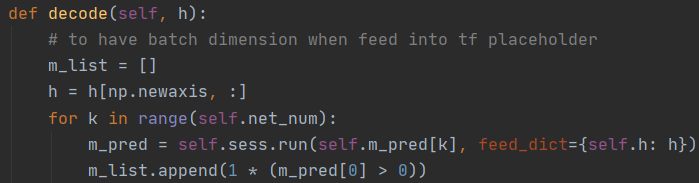note： 出现这种错误是唯独不匹配导致的错误，这个错误的根源要知道。

# ; 2 解决方法

## 2.2 修改程序使其匹配

image = tf.reshape(image_data, [1, 32, 32, 3])

# file_label = np.sum(file_label)

image形状为(64,64,3)。

image = array(img).reshape(1,64,64,3)

# 3 其他报错

##### 3.1 报错：Ignore above cudart dlerror if you do not have a GPU set up on your machine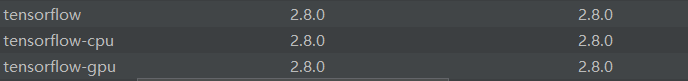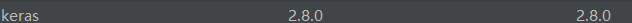##### ; 3.2 报错：AttributeError: module 'tensorflow' has no attribute 'reset_default_graph'

`tf.reset_default_graph()`改为：

``````tf.compat.v1.reset_default_graph()
``````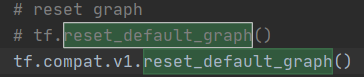`````` import tensorflow as tf
tf.compat.v1.reset_default_graph()
``````

##### 3.3 报错：AttributeError: module 'tensorflow' has no attribute 'placeholder'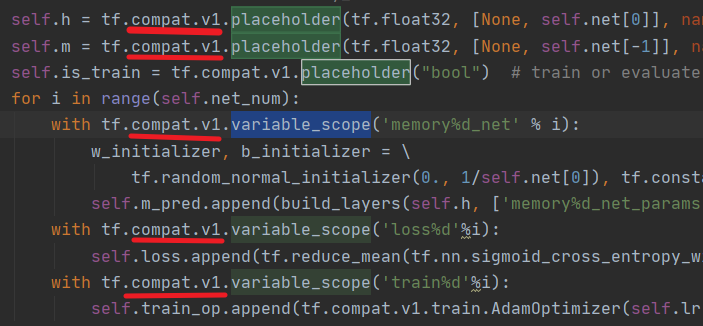Y=tf.compat.v1.placeholder("float")

##### ; 3.4 报错：ValueError: Cannot feed value of shape (1, 6) for Tensor h:0, which has shape (None, 7)

Original: https://blog.csdn.net/chengxuyuan99/article/details/123872411
Author: 小瑞o
Title: 【已解决】ValueError: Cannot feed value of shape (1, 6) for Tensor h:0, which has shape (None, 7)涉及其他报错问题